# Resources tagged with: Working systematically

Filter by: Content type:
Age range:
Challenge level:

### There are 127 results

Broad Topics > Using, Applying and Reasoning about Mathematics > Working systematically### The Great Weights Puzzle

##### Age 14 to 16 Challenge Level:

You have twelve weights, one of which is different from the rest. Using just 3 weighings, can you identify which weight is the odd one out, and whether it is heavier or lighter than the rest?### All-variables Sudoku

##### Age 11 to 18 Challenge Level:

The challenge is to find the values of the variables if you are to solve this Sudoku.### Special Numbers

##### Age 11 to 14 Challenge Level:

My two digit number is special because adding the sum of its digits to the product of its digits gives me my original number. What could my number be?### Advent Calendar 2011 - Secondary

##### Age 11 to 18 Challenge Level:

Advent Calendar 2011 - a mathematical activity for each day during the run-up to Christmas.### A Long Time at the Till

##### Age 14 to 18 Challenge Level:

Try to solve this very difficult problem and then study our two suggested solutions. How would you use your knowledge to try to solve variants on the original problem?### Summing Consecutive Numbers

##### Age 11 to 14 Challenge Level:

15 = 7 + 8 and 10 = 1 + 2 + 3 + 4. Can you say which numbers can be expressed as the sum of two or more consecutive integers?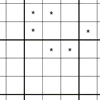##### Age 11 to 14 Challenge Level:

You need to find the values of the stars before you can apply normal Sudoku rules.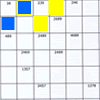### Intersection Sudoku 1

##### Age 11 to 16 Challenge Level:

A Sudoku with a twist.### An Introduction to Magic Squares

##### Age 7 to 16### Problem Solving, Using and Applying and Functional Mathematics

##### Age 5 to 18 Challenge Level:

Problem solving is at the heart of the NRICH site. All the problems give learners opportunities to learn, develop or use mathematical concepts and skills. Read here for more information.### Consecutive Negative Numbers

##### Age 11 to 14 Challenge Level:

Do you notice anything about the solutions when you add and/or subtract consecutive negative numbers?### Games Related to Nim

##### Age 5 to 16

This article for teachers describes several games, found on the site, all of which have a related structure that can be used to develop the skills of strategic planning.##### Age 11 to 16 Challenge Level:

Four numbers on an intersection that need to be placed in the surrounding cells. That is all you need to know to solve this sudoku.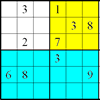### Colour Islands Sudoku

##### Age 11 to 14 Challenge Level:

An extra constraint means this Sudoku requires you to think in diagonals as well as horizontal and vertical lines and boxes of nine.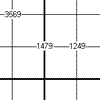##### Age 11 to 16 Challenge Level:

This is a variation of sudoku which contains a set of special clue-numbers. Each set of 4 small digits stands for the numbers in the four cells of the grid adjacent to this set.##### Age 7 to 14 Challenge Level:

I added together some of my neighbours' house numbers. Can you explain the patterns I noticed?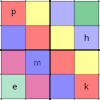### Simultaneous Equations Sudoku

##### Age 11 to 16 Challenge Level:

Solve the equations to identify the clue numbers in this Sudoku problem.### Where Can We Visit?

##### Age 11 to 14 Challenge Level:

Charlie and Abi put a counter on 42. They wondered if they could visit all the other numbers on their 1-100 board, moving the counter using just these two operations: x2 and -5. What do you think?### Window Frames

##### Age 5 to 14 Challenge Level:

This task encourages you to investigate the number of edging pieces and panes in different sized windows.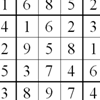### Twin Chute-swapping Sudoku

##### Age 14 to 18 Challenge Level:

A pair of Sudokus with lots in common. In fact they are the same problem but rearranged. Can you find how they relate to solve them both?### Warmsnug Double Glazing

##### Age 14 to 16 Challenge Level:

How have "Warmsnug" arrived at the prices shown on their windows? Which window has been given an incorrect price?### Plum Tree

##### Age 14 to 18 Challenge Level:

Label this plum tree graph to make it totally magic!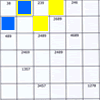### Intersection Sudoku 2

##### Age 11 to 16 Challenge Level:

A Sudoku with a twist.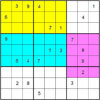### Colour Islands Sudoku 2

##### Age 11 to 18 Challenge Level:

In this Sudoku, there are three coloured "islands" in the 9x9 grid. Within each "island" EVERY group of nine cells that form a 3x3 square must contain the numbers 1 through 9.### Maths Trails

##### Age 7 to 14

The NRICH team are always looking for new ways to engage teachers and pupils in problem solving. Here we explain the thinking behind maths trails.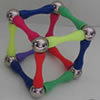### Magnetic Personality

##### Age 7 to 16 Challenge Level:

60 pieces and a challenge. What can you make and how many of the pieces can you use creating skeleton polyhedra?### Tetrahedra Tester

##### Age 11 to 14 Challenge Level:

An irregular tetrahedron is composed of four different triangles. Can such a tetrahedron be constructed where the side lengths are 4, 5, 6, 7, 8 and 9 units of length?### Product Sudoku

##### Age 11 to 14 Challenge Level:

The clues for this Sudoku are the product of the numbers in adjacent squares.### American Billions

##### Age 11 to 14 Challenge Level:

Play the divisibility game to create numbers in which the first two digits make a number divisible by 2, the first three digits make a number divisible by 3...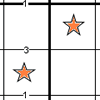### Constellation Sudoku

##### Age 14 to 18 Challenge Level:

Special clue numbers related to the difference between numbers in two adjacent cells and values of the stars in the "constellation" make this a doubly interesting problem.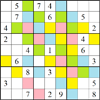### Rainstorm Sudoku

##### Age 14 to 16 Challenge Level:

Use the clues about the shaded areas to help solve this sudoku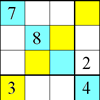### Rectangle Outline Sudoku

##### Age 11 to 16 Challenge Level:

Each of the main diagonals of this sudoku must contain the numbers 1 to 9 and each rectangle width the numbers 1 to 4.### Squares in Rectangles

##### Age 11 to 14 Challenge Level:

A 2 by 3 rectangle contains 8 squares and a 3 by 4 rectangle contains 20 squares. What size rectangle(s) contain(s) exactly 100 squares? Can you find them all?##### Age 11 to 14 Challenge Level:

Replace the letters with numbers to make the addition work out correctly. R E A D + T H I S = P A G E### Football Sum

##### Age 11 to 14 Challenge Level:

Find the values of the nine letters in the sum: FOOT + BALL = GAME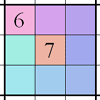### Integrated Product Sudoku

##### Age 11 to 16 Challenge Level:

This Sudoku puzzle can be solved with the help of small clue-numbers on the border lines between pairs of neighbouring squares of the grid.### Sticky Numbers

##### Age 11 to 14 Challenge Level:

Can you arrange the numbers 1 to 17 in a row so that each adjacent pair adds up to a square number?##### Age 11 to 14 Challenge Level:

If you take a three by three square on a 1-10 addition square and multiply the diagonally opposite numbers together, what is the difference between these products. Why?### Number Daisy

##### Age 11 to 14 Challenge Level:

Can you find six numbers to go in the Daisy from which you can make all the numbers from 1 to a number bigger than 25?### Two and Two

##### Age 11 to 14 Challenge Level:

How many solutions can you find to this sum? Each of the different letters stands for a different number.### LCM Sudoku II

##### Age 11 to 18 Challenge Level:

You are given the Lowest Common Multiples of sets of digits. Find the digits and then solve the Sudoku.### Cayley

##### Age 11 to 14 Challenge Level:

The letters in the following addition sum represent the digits 1 ... 9. If A=3 and D=2, what number is represented by "CAYLEY"?### M, M and M

##### Age 11 to 14 Challenge Level:

If you are given the mean, median and mode of five positive whole numbers, can you find the numbers?### 9 Weights

##### Age 11 to 14 Challenge Level:

You have been given nine weights, one of which is slightly heavier than the rest. Can you work out which weight is heavier in just two weighings of the balance?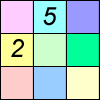### Difference Sudoku

##### Age 14 to 16 Challenge Level:

Use the differences to find the solution to this Sudoku.### A First Product Sudoku

##### Age 11 to 14 Challenge Level:

Given the products of adjacent cells, can you complete this Sudoku?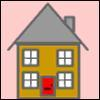### Smith and Jones

##### Age 14 to 16 Challenge Level:

Mr Smith and Mr Jones are two maths teachers. By asking questions, the answers to which may be right or wrong, Mr Jones is able to find the number of the house Mr Smith lives in... Or not!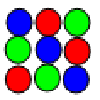### Latin Squares

##### Age 11 to 18

A Latin square of order n is an array of n symbols in which each symbol occurs exactly once in each row and exactly once in each column.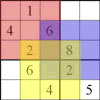### Seasonal Twin Sudokus

##### Age 11 to 16 Challenge Level:

This pair of linked Sudokus matches letters with numbers and hides a seasonal greeting. Can you find it?### Factor Lines

##### Age 7 to 14 Challenge Level:

Arrange the four number cards on the grid, according to the rules, to make a diagonal, vertical or horizontal line.